## 2.2 Unipart network for seed lots analysis

This section deals with unipart network that represents the relationships between seed lots.

### 2.2.1 Steps with PPBstats

• Format the data with format_data_PPBstats()
• get descriptive plot with plot()

### 2.2.2 Format the data

The format required is a data frame with the following compulsory columns as factor:

• "seed_lot_parent" : name of the parent seed lot in the relationship
• "seed_lot_child" ; name of the child seed lots in the relationship
• "relation_type" : the type of relationship between the seed lots
• "relation_year_start" : the year when the relationship starts
• "relation_year_end" : the year when the relationship stops
• "germplasm_parent" : the germplasm associated to the parent seed lot
• "location_parent" : the location associated to the parent seed lot
• "year_parent" : the year of the last relationship of the parent seed lot
• "germplasm_child" : the germplasm associated to the child seed lot
• "location_child" : the location associated to the child seed lot
• "year_child" : represents the year of the last relation event of the child seed lot

Possible options are : "long_parent", "lat_parent", "long_child", "lat_child" to get map representation, supplementary variables with tags: "_parent", "_child" or "_relation".

The format of the data are checked by the function format_data_PPBstats() with the following arguments :

• type : "data_network"
• network_part : "unipart"
• vertex_type : "seed_lots"

The function returns list of igraph object1 coming from igraph::graph_from_data_frame().

data(data_network_unipart_sl)
head(data_network_unipart_sl)
##          seed_lot_parent         seed_lot_child relation_type
## 1 germ-8_loc-1_2007_0001 germ-8_loc-1_2008_0001     selection
## 2 germ-8_loc-1_2008_0001 germ-8_loc-1_2009_0001  reproduction
## 3 germ-8_loc-1_2009_0001 germ-8_loc-2_2009_0001     diffusion
## 4 germ-8_loc-1_2008_0001 germ-8_loc-1_2009_0001     selection
## 5 germ-1_loc-1_2005_0001 germ-8_loc-1_2006_0001  reproduction
## 6 germ-6_loc-1_2005_0001 germ-8_loc-1_2006_0001  reproduction
##   relation_year_start relation_year_end germplasm_parent location_parent
## 1                2007              2008           germ-8           loc-1
## 2                2008              2009           germ-8           loc-1
## 3                2009              2009           germ-8           loc-1
## 4                2008              2009           germ-8           loc-1
## 5                2005              2006           germ-1           loc-1
## 6                2005              2006           germ-6           loc-1
##   year_parent alt_parent long_parent lat_parent germplasm_child
## 1        2007         50    0.616363   44.20314          germ-8
## 2        2008         50    0.616363   44.20314          germ-8
## 3        2009         50    0.616363   44.20314          germ-8
## 4        2008         50    0.616363   44.20314          germ-8
## 5        2005         50    0.616363   44.20314          germ-8
## 6        2005         50    0.616363   44.20314          germ-8
##   location_child year_child alt_child long_child lat_child
## 1          loc-1       2008        50   0.616363  44.20314
## 2          loc-1       2009        50   0.616363  44.20314
## 3          loc-2       2009       360   3.087025  45.77722
## 4          loc-1       2009        50   0.616363  44.20314
## 5          loc-1       2006        50   0.616363  44.20314
## 6          loc-1       2006        50   0.616363  44.20314
net_unipart_sl = format_data_PPBstats(
type = "data_network",
data = data_network_unipart_sl,
network_part = "unipart",
vertex_type =  "seed_lots")
## data has been formated for PPBstats functions.
length(net_unipart_sl)
##  1
head(net_unipart_sl)
## []
## IGRAPH 874b15c DN-- 81 94 --
## + attr: name (v/c), germplasm (v/c), location (v/c), year (v/c),
## | alt (v/c), long (v/c), lat (v/c), format (v/c), relation_type
## | (e/c)
## + edges from 874b15c (vertex names):
##  germ-8_loc-1_2007_0001->germ-8_loc-1_2008_0001
##  germ-8_loc-1_2008_0001->germ-8_loc-1_2009_0001
##  germ-8_loc-1_2009_0001->germ-8_loc-2_2009_0001
##  germ-8_loc-1_2008_0001->germ-8_loc-1_2009_0001
##  germ-1_loc-1_2005_0001->germ-8_loc-1_2006_0001
##  germ-6_loc-1_2005_0001->germ-8_loc-1_2006_0001
## + ... omitted several edges

### 2.2.3 Describe the data

The different representations are done with the plot() function.

For network representation, set plot_type = "network" diffusion event are displayed with a curve. in_col can be settled to customize color of vertex.

p_net = plot(net_unipart_sl, plot_type = "network", in_col = "location")
p_net
## []
## []$network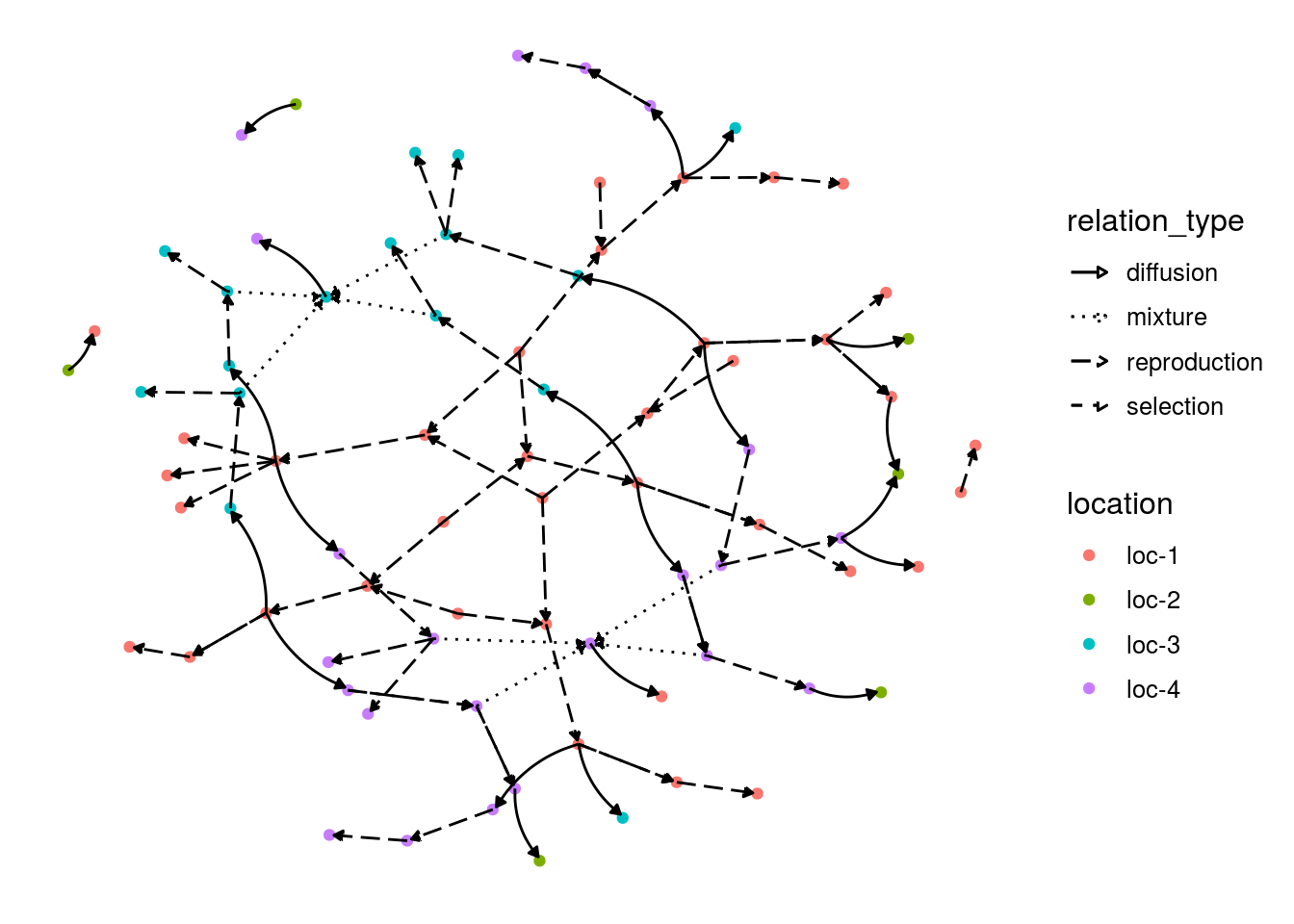In order to get the network organized in a chronologiical order and by location, set organize_sl = TRUE. This representation is possible if the seed lots are under the following format : GERMPLASM_LOCATION_YEAR_DIGIT. p_net_org = plot(net_unipart_sl, plot_type = "network", organize_sl = TRUE) p_net_org ## [] ## []$network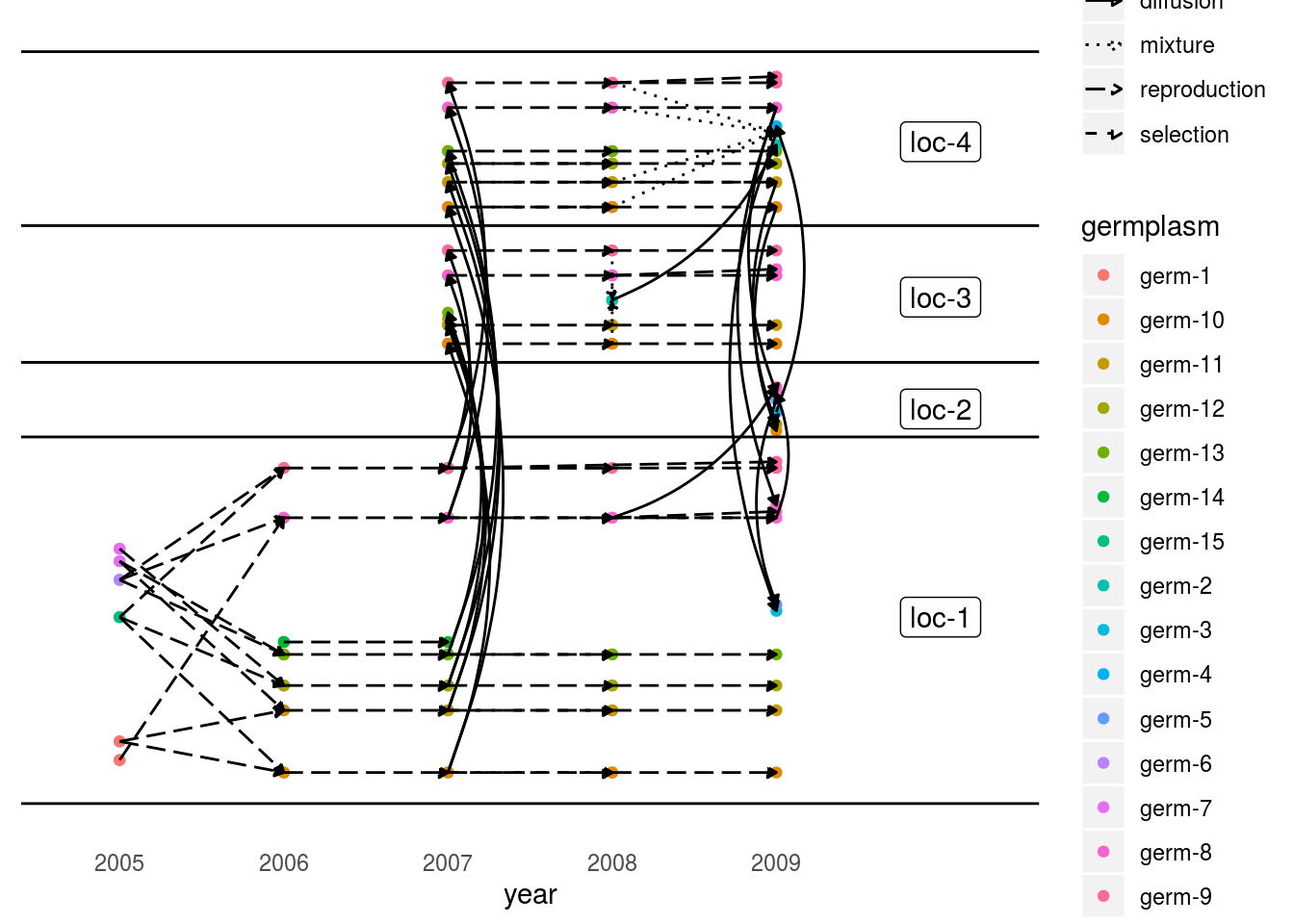To have information on the seed lots that are represented, plot_type = "barplot" can be used. Choose what to represent on the x axis and in color as well as the number of parameter per plot.

p_bar = plot(net_unipart_sl, plot_type = "barplot", in_col = "location",
x_axis = "germplasm", nb_parameters_per_plot_x_axis = 5,
nb_parameters_per_plot_in_col = 5)
p_bar[]$barplot$germplasm-1|location-1 # first element of the plot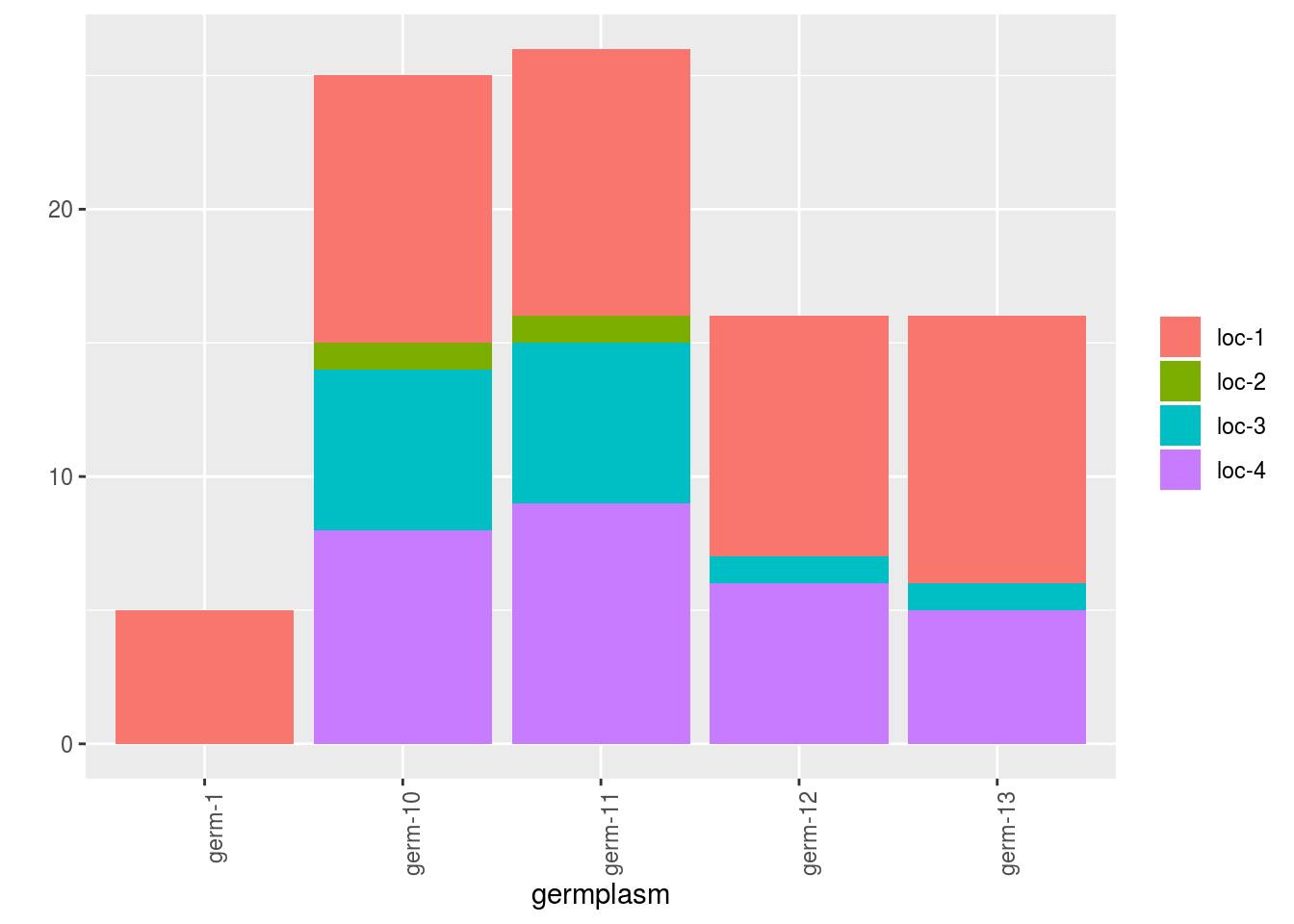Barplot can also be use to study the relation within the network. The name of the relation must be put in the argument vec_variables. The results is a list of two elements for each variable:

• nb_received: number of seed lots that end the relation
• nb_given: number of seed lots that start the relation
p_bar = plot(net_unipart_sl, plot_type = "barplot", vec_variables = "diffusion",
nb_parameters_per_plot_x_axis = 100, x_axis = "location", in_col = "year")
p_bar
## []
## []$diffusion ## []$diffusion$nb_received ## []$diffusion$nb_received$location-1|year-1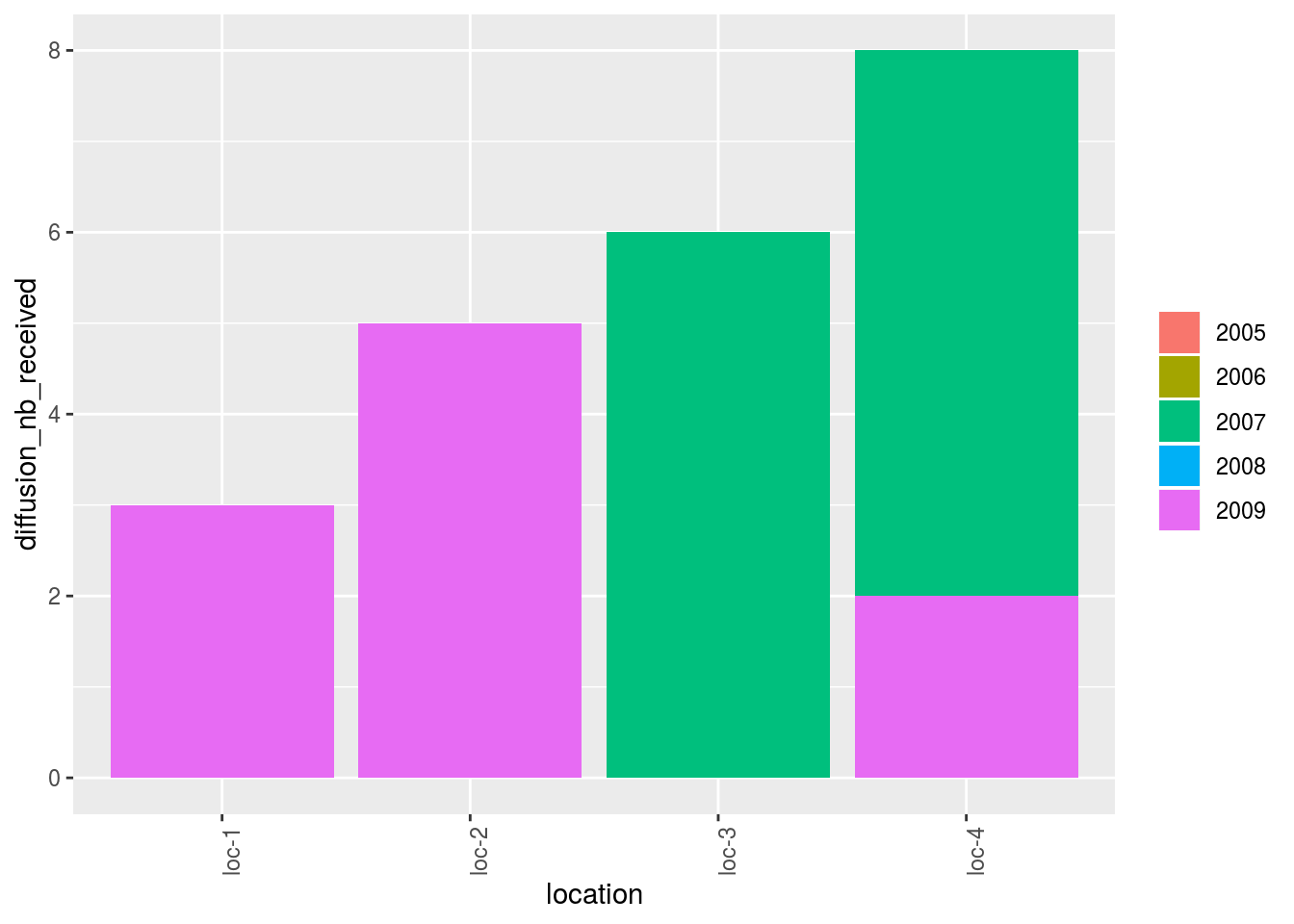##
##
## []$diffusion$nb_given
## []$diffusion$nb_given$location-1|year-1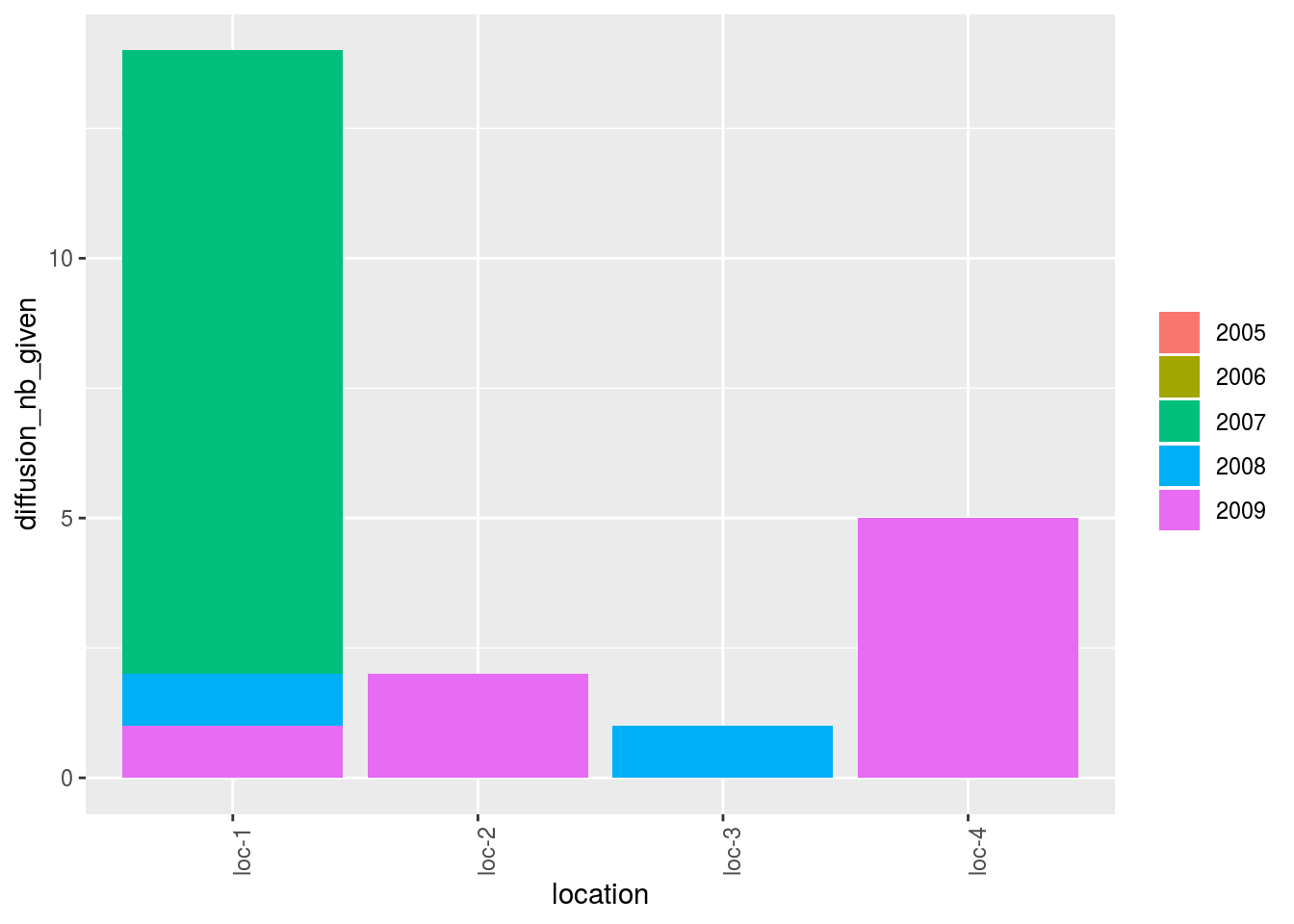Location present on the network can be displayed on a map with plot_type = "map". When using map, do not forget to use credit : Map tiles by Stamen Design, under CC BY 3.0. Data by OpenStreetMap, under ODbL. p_map = plot(net_unipart_sl, plot_type = "map", labels_on = "location") p_map ## [] ## []$map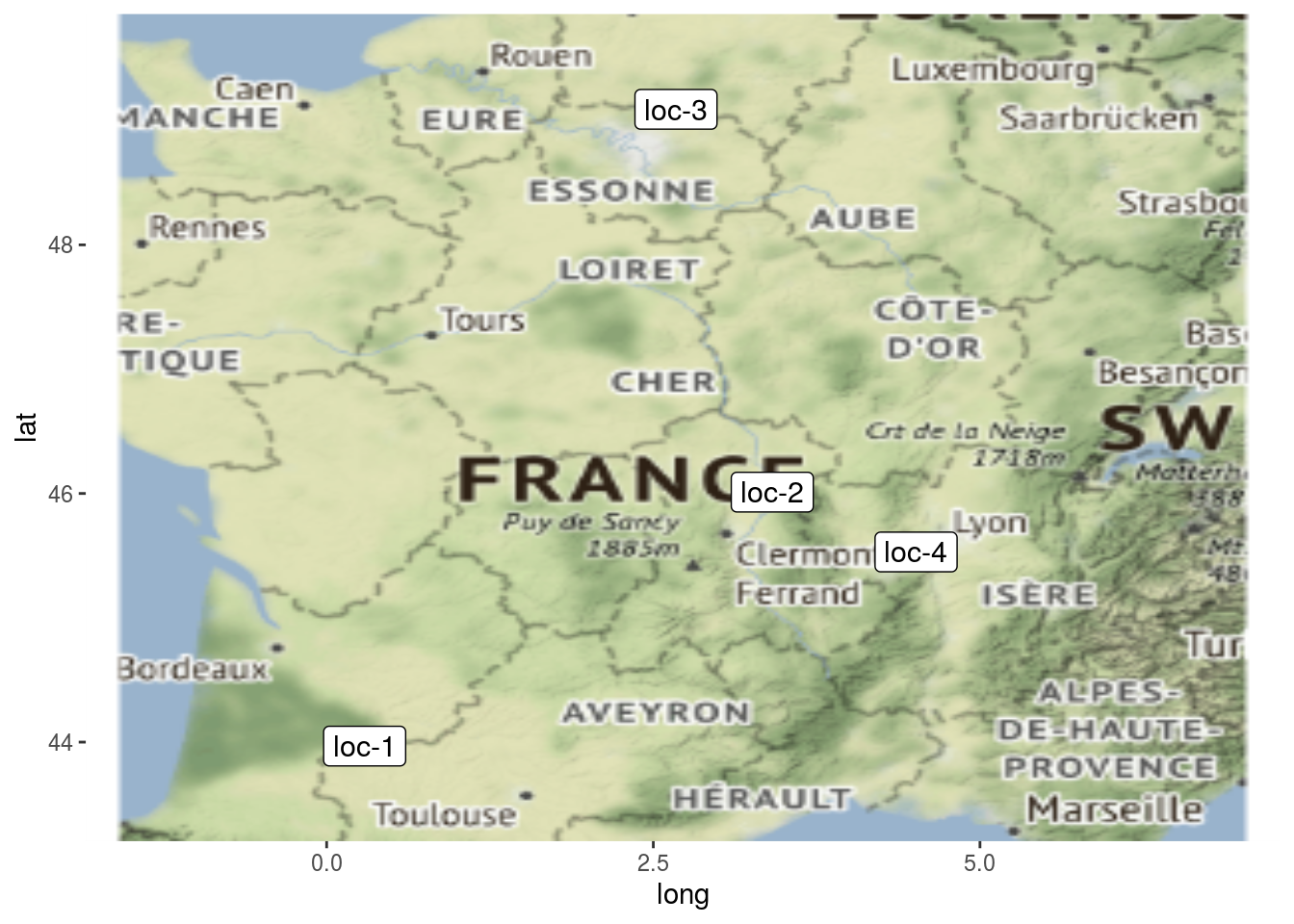It can be interesting to plot information regarding a variable on map with a pie with plot_type = "map" and by setting arguments data_to_pie and variable:

nb_values = 30
data_to_pie = data.frame(
seed_lot = rep(c("germ-4_loc-4_2009_0001", "germ-9_loc-4_2009_0001", "germ-10_loc-3_2009_0001", "germ-12_loc-3_2007_0001", "germ-11_loc-2_2009_0001", "germ-10_loc-2_2009_0001"), each = nb_values),
location = rep(c("loc-1", "loc-1", "loc-3", "loc-3", "loc-2", "loc-2"), each = nb_values),
year = rep(c("2009", "2008", "2007", "2007", "2009", "2009"), each = nb_values),
germplasm = rep(c("germ-7", "germ-2", "germ-6", "germ-4", "germ-5", "germ-13"), each = nb_values),
block = 1,
X = 1,
Y = 1,
y1 = rnorm(nb_values*6, 10, 2), # quanti
y2 = rep(c("cat1", "cat1", "cat2", "cat3", "cat3", "cat4"), each = nb_values)  # quali
)

data_to_pie$seed_lot = as.factor(as.character(data_to_pie$seed_lot))
data_to_pie$location = as.factor(as.character(data_to_pie$location))
data_to_pie$year = as.factor(as.character(data_to_pie$year))
data_to_pie$germplasm = as.factor(as.character(data_to_pie$germplasm))
data_to_pie$block = as.factor(as.character(data_to_pie$block))
data_to_pie$X = as.factor(as.character(data_to_pie$X))
data_to_pie$Y = as.factor(as.character(data_to_pie$Y))

data_to_pie = format_data_PPBstats(data_to_pie, type = "data_agro")
## data has been formated for PPBstats functions.
# y1 is a quantitative variable
p_map_pies_y1 = plot(net_unipart_sl, data_to_pie, plot_type = "map", vec_variables = "y1")
p_map_pies_y1
## []
## []$y1_map_with_pies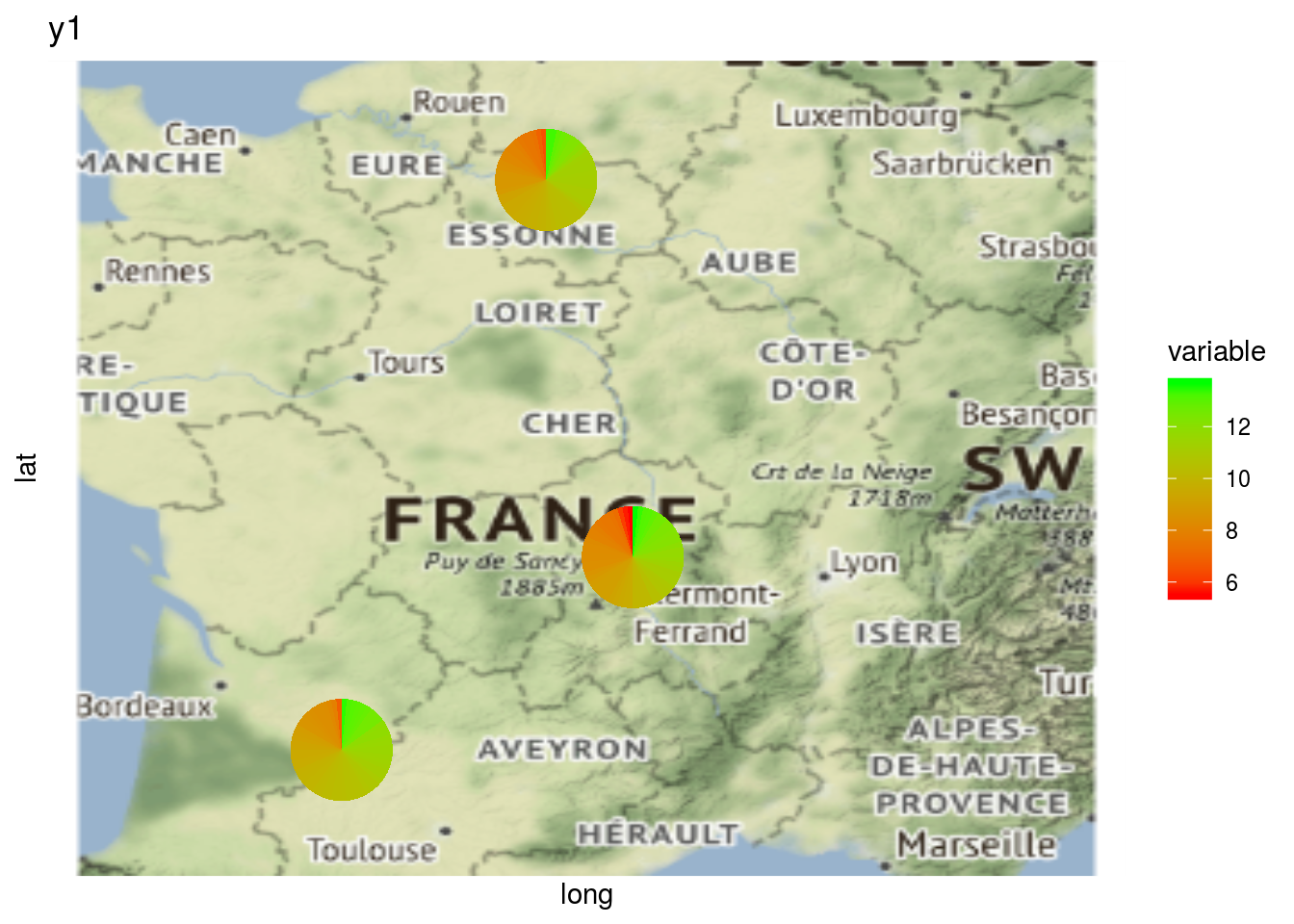# y2 is a qualitative variable p_map_pies_y2 = plot(net_unipart_sl, data_to_pie, plot_type = "map", vec_variables = "y2") p_map_pies_y2 ## [] ## []$y2_map_with_pies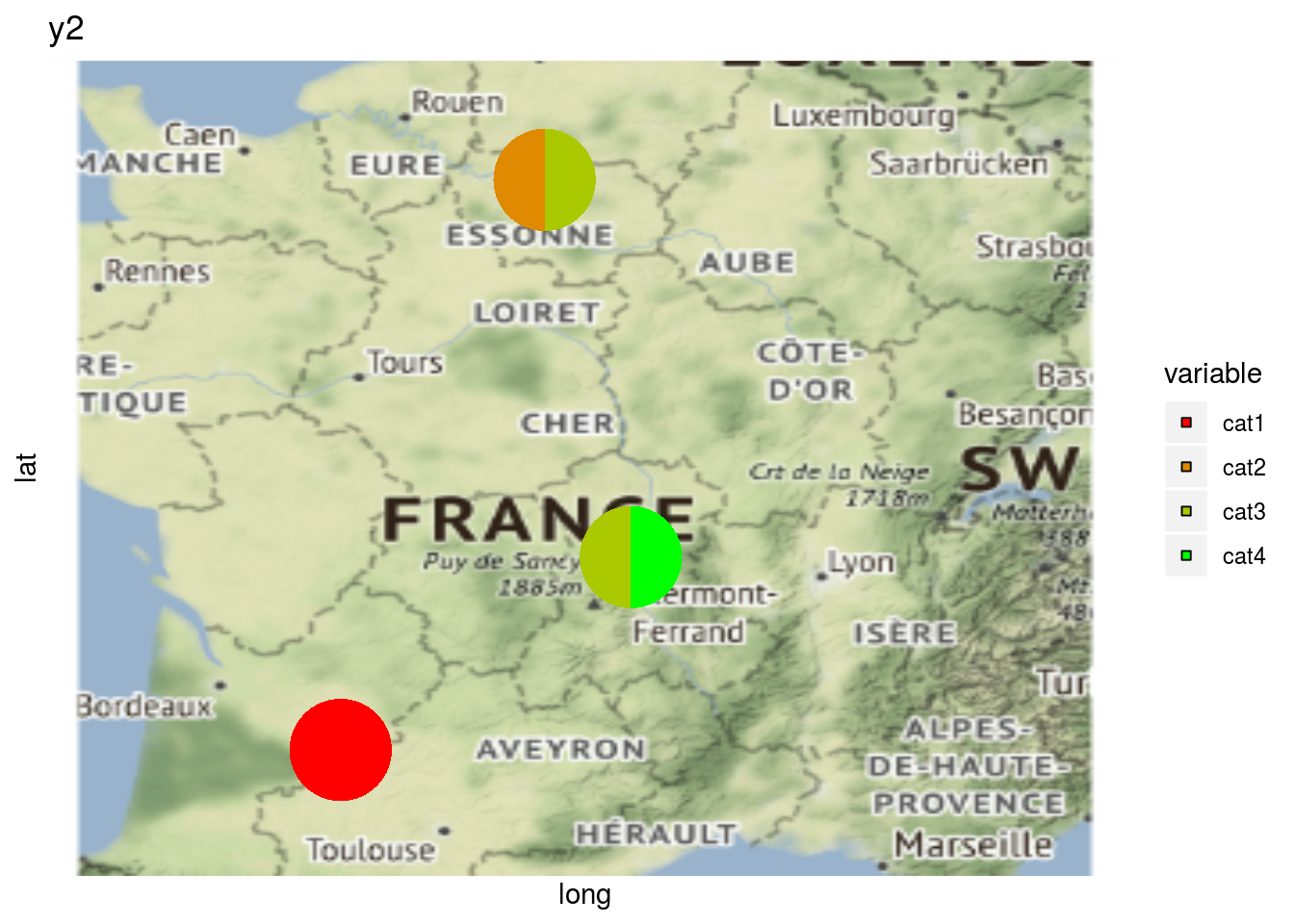or on the network with a pie with plot_type = "network" and by setting arguments data_to_pie and vec_variables:

# y1 is a quantitative variable
p_net_pies_y1 = plot(net_unipart_sl, data_to_pie, plot_type = "network", vec_variables = "y1")
p_net_pies_y1
## []
## []$y1_network_with_pies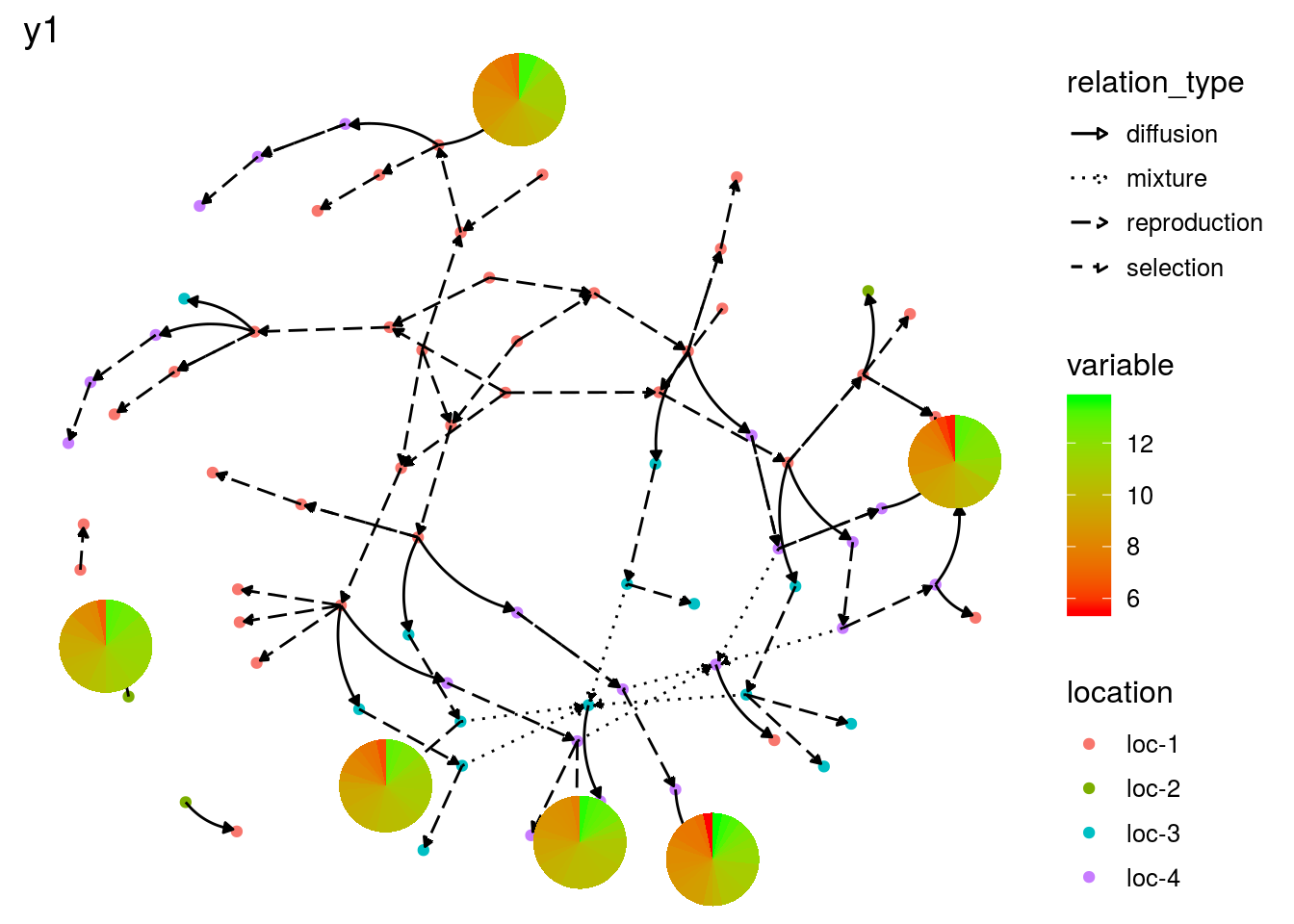# y2 is a qualitative variable p_net_pies_y2 = plot(net_unipart_sl, data_to_pie, plot_type = "network", vec_variables = "y2") p_net_pies_y2 ## [] ## []$y2_network_with_pies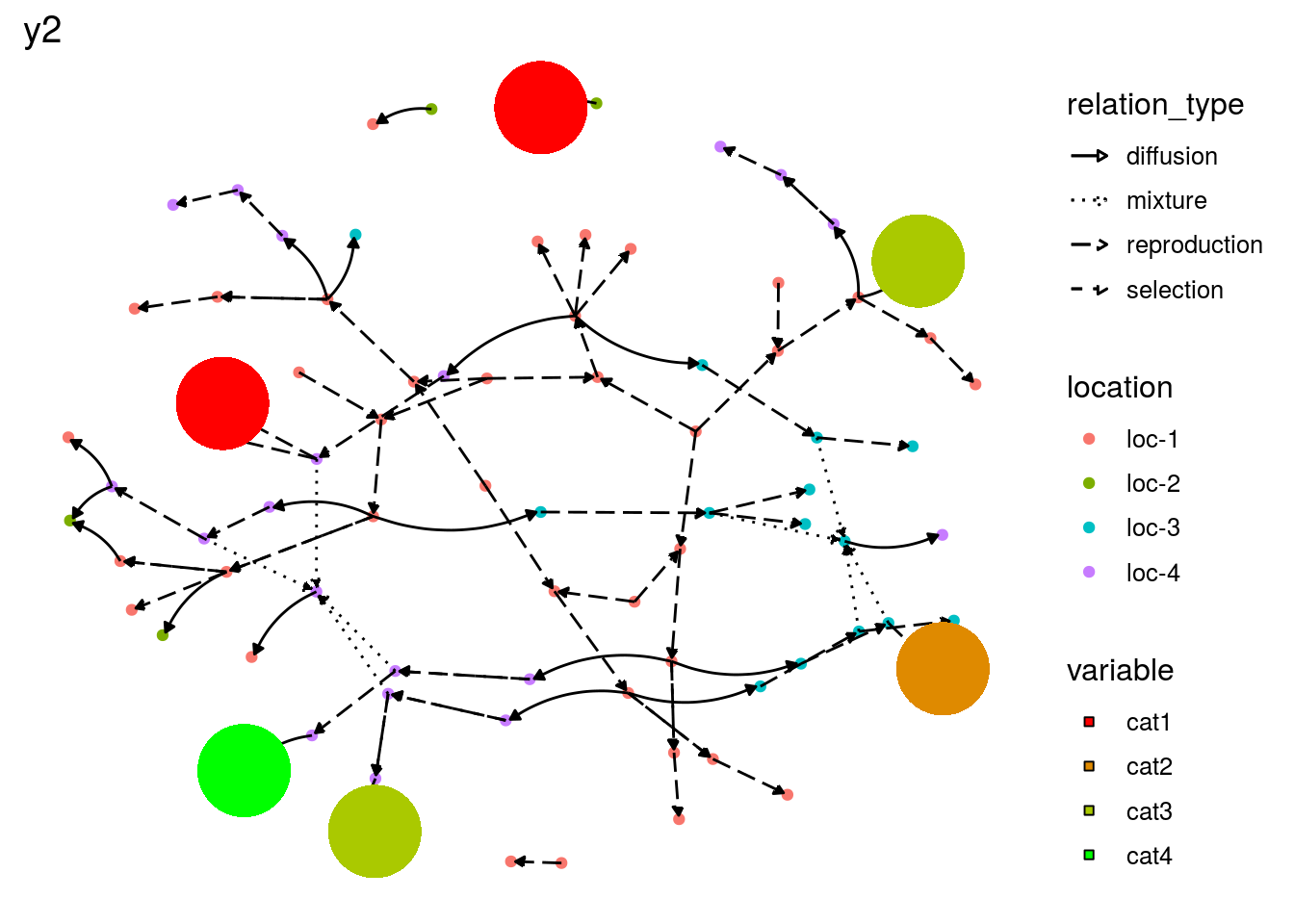The same can be done regarding relation type of the network. This can be displayed on a map but not on a network.

p_map_pies_diff = plot(net_unipart_sl, plot_type = "map", vec_variables = "diffusion")
p_map_pies_diff
## []
## []$diffusion_nb_received_map_with_pies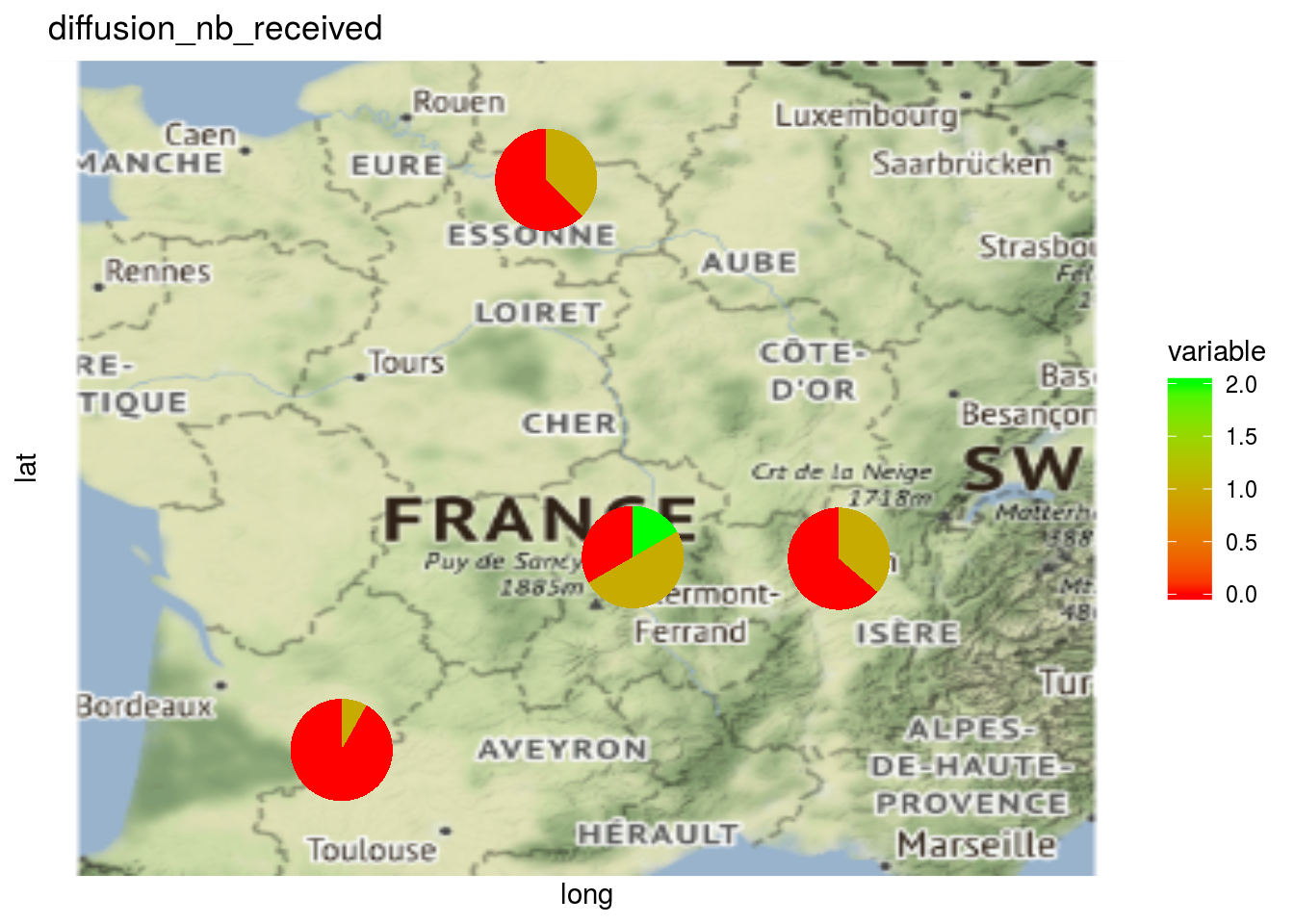## ## []$diffusion_nb_given_map_with_pies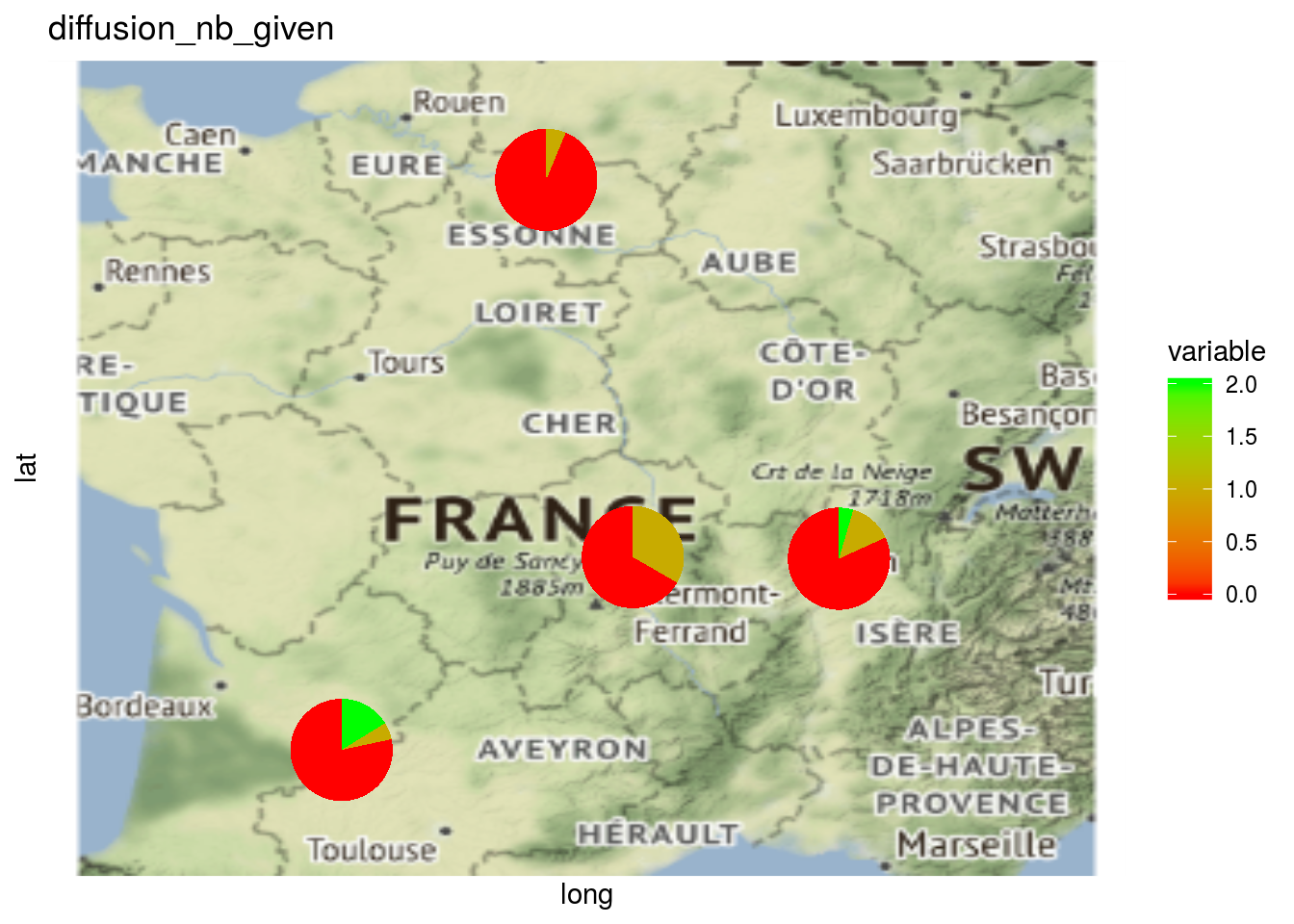Here the pies represent the repartition of the number of seed lots.